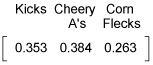##### Finite Math For Dummies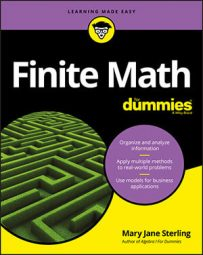If your finite math instructor asks you to predict the likelihood of an action repeating over time, you may need to use a transition matrix to do this. A transition matrix consists of a square matrix that gives the probabilities of different states going from one to another.

With a transition matrix, you can perform matrix multiplication and determine trends, if there are any, and make predications.

Consider the table showing the purchasing patterns involving different cereals. You see all the percentages showing the probability of going from one state to another, but which of the cereals does the consumer actually end up buying most frequently in the long run?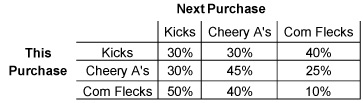One way to look at continued purchasing is to create a tree diagram. In the following figure, you see two consecutive “rounds” of purchases.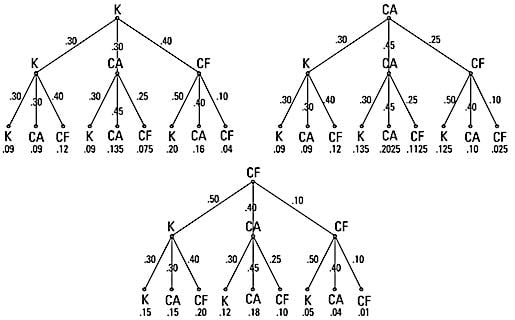Which kind of cereal will the consumer buy?

If you want the probability that the consumer purchases Kicks first, tries it again or something else, and then purchases Kicks the next time, add up the , , and branches: , or 38% of the time. If you want the probability that the consumer purchases Cheery A’s first, tries something else or repeats Cheery A’s, and then tries Corn Flecks, add up the , , and branches. This comes out to , or almost 26% of the time.

The tree is helpful in that it shows you what the choices are and how the percentages work in determining patterns, but there’s a much easier and neater way to compute these values.

To perform computations and study this further, create a transition matrix, referring back to the chart showing purchases and using the decimal values of the percentages. Name it matrix C.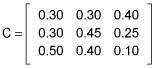Next, use matrix multiplication to find C². As a quick hint, when multiplying matrices, you find the element in the first row, first column of the product, labeled c11, when you multiply the elements in the first row of the first matrix times the corresponding elements in the first column of the second matrix and then add up the products.

In a matrix A, the element in the nth row, kth column is labeled ank.

The element in the first row and second column of the product, c12, uses the elements in the first row of the first matrix and second column of the second matrix, and so on for the rest of the elements.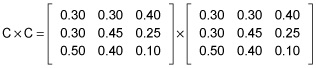So, you take the first row of the left matrix times the first column of the second matrix to get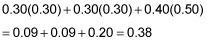Yes. This is the same computation as was done using the tree to find the probability that a consumer starting with Kicks would return to it in two more purchases.

Performing the matrix multiplication, you have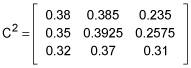Continuing this multiplication process, by the time C6 appears (the chances of buying a particular cereal at the fifth purchase time after the initial purchase), a pattern emerges.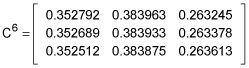Notice that the numbers in each column round to the same three decimal places. This is going to become even clearer, using higher powers of C, until some nth matrix power becomes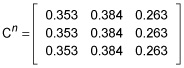The matrix shows you the pattern or trend.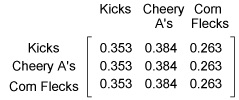No matter which cereal the consumer bought first, in the long run there’s a 35.3% chance that she’ll purchase Kicks, a 38.4% chance that she’ll purchase Cheery A’s, and a 26.3% chance that she’ll purchase Corn Flecks. This transition matrix has reached an equilibrium, where it won’t change with more repeated multiplication. You can write this situation with a single-line matrix: